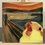# Navier-Stokes and Blood.

Let us assume that blood moves only along the blood vessel, by which we mean that there is no radial or angular components in the speed of the blood. If we consider a straight blood vessel, then, the speed will be written as

$\mathbf{u}(r,z) = u(r,z)\mathbf{\widehat{z}},$

where there is no angular dependence considering the symmetry of the problem. If we consider a steady flow, then Navier-Stokes equation (NVE) in cylindrical coordinates will give us

$0 = -\nabla p + \mu \nabla^2\mathbf{u} = -\nabla p + \frac{\mu}{r} \frac{\partial }{\partial r} \left ( r \frac{\partial u(r,z)}{\partial r} \right ) + \frac{\partial^2 u(r,z)}{\partial z^2},$

where $p$ is a function denoting the pressure field. Let us assume that blood pressure drops from arterial pressure $p_0$ by an amount $\Delta$ after traveling a distance $L$ in a linear fashion:

$p(z) = p_0 - \frac{\Delta}{L} z \;\;\ \Rightarrow \;\;\ \nabla p = - \frac{\Delta}{L} \equiv q.$

Therefore NVE yields:

$-q + \frac{\mu}{r} \frac{\partial }{\partial r} \left ( r \frac{\partial u(r,z)}{\partial r} \right ) + \frac{\partial^2 u(r,z)}{\partial z^2} = 0 \;\;\; \left [ 1 \right ].$

But, as we know, mass must be conserved. So

$\nabla \cdot \mathbf{u} = \frac{\partial u}{\partial z} = 0 \;\;\; \Rightarrow \;\;\; u(r,z) = C_1 + f(r),$

where $C_1$ is a constant and $f(r)$ is a function of the distance to the center of the flow, only. As there is no dependence in $z,$ the second partial derivative in relation to this component must be $0$ and equation $\left [ 1 \right ]$ reduces to

$-q + \frac{\mu}{r} \frac{\partial }{\partial r} \left ( r \frac{\partial f}{\partial r} \right ) = 0,$

which easily solves to

$f(r) = \frac{qr^2}{4\mu} + C_2 \ln(r) + C_3 \;\;\; \therefore \;\;\; u(r,z) \equiv u(r) = \frac{qr^2}{4\mu} + C_2 \ln(r) + C_3 + C_1 \equiv \frac{qr^2}{4\mu} + C,$

where we've taken $C_2 = 0$ because we want the function to not diverge as $r \rightarrow 0$ and $C_1 + C_3 = C.$ If the speed function is required to be smooth everywhere, one would like that $u(R) = 0,$ where $R$ is the radius of the blood vessel. This condition settles a value for $C$ and one can find the unique solution:

$u(r) = \frac{qR^2}{4\mu} \left ( \frac{r^2}{R^2} - 1 \right ).$

As one can see, even though it might seem counterintuitive, in a steady flow regime the speed of the flow in a linear-gradient pressure drop does not depend on the distance from the beginning of the pressure drop.Note by Lucas Tell Marchi
3 months, 1 week ago

This discussion board is a place to discuss our Daily Challenges and the math and science related to those challenges. Explanations are more than just a solution — they should explain the steps and thinking strategies that you used to obtain the solution. Comments should further the discussion of math and science.

When posting on Brilliant:

• Use the emojis to react to an explanation, whether you're congratulating a job well done , or just really confused .
• Ask specific questions about the challenge or the steps in somebody's explanation. Well-posed questions can add a lot to the discussion, but posting "I don't understand!" doesn't help anyone.
• Try to contribute something new to the discussion, whether it is an extension, generalization or other idea related to the challenge.

MarkdownAppears as
*italics* or _italics_ italics
**bold** or __bold__ bold
- bulleted- list
• bulleted
• list
1. numbered2. list
1. numbered
2. list
Note: you must add a full line of space before and after lists for them to show up correctly
paragraph 1paragraph 2

paragraph 1

paragraph 2

[example link](https://brilliant.org)example link
> This is a quote
This is a quote
    # I indented these lines
# 4 spaces, and now they show
# up as a code block.

print "hello world"
# I indented these lines
# 4 spaces, and now they show
# up as a code block.

print "hello world"
MathAppears as
Remember to wrap math in $$ ... $$ or $ ... $ to ensure proper formatting.
2 \times 3 $2 \times 3$
2^{34} $2^{34}$
a_{i-1} $a_{i-1}$
\frac{2}{3} $\frac{2}{3}$
\sqrt{2} $\sqrt{2}$
\sum_{i=1}^3 $\sum_{i=1}^3$
\sin \theta $\sin \theta$
\boxed{123} $\boxed{123}$